NCERT Exemplar Class 6 Science Chapter 10 Motion and Measurement of Distances are part of NCERT Exemplar Class 6 Science. Here we have given NCERT Exemplar Class 6 Science Solutions Chapter 10 Motion and Measurement of Distances.

## NCERT Exemplar Class 6 Science Solutions Chapter 10 Motion and Measurement of Distances

Multiple Choice Questions

Question 1.
The distance between Delhi and Mumbai is usually expressed in units of
(a) decametre
(b) metre
(c) centimetre
(d) kilometre
Solution:
(d) : The distance between Delhi and Mumbai is very large so it is usually expressed in units of kilometre.

Question 2.
Which of the following does not express a time interval?
(a) A day
(b) A second
(c) A school period
(d) Time of the first bell in the school
Solution:
(d): Time of the first bell in the school represents a point of time, not a time interval.

Question 3.
Figure shows a measuring scale which is usually supplied with a geometry box. Which of the following distance cannot be measured with this scale by using it only once?(a) 0.1m
(b) 0.15 m
(c) 0.2 m
(d) 0.05 m
Solution:
(c): This scale is 0.15 m long so by using it only once, 0.2 m cannot be measured.

Question 4.
A piece of ribbon folded five times is placed along a 30 cm long measuring scale as shown in figure.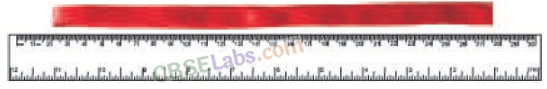The length of the ribbon is between
(a) 1.15 m – 1.25 m
(b) 1.25 m – 1.35 m
(c) 1.50 m – 1.60 m
(d) 1.60 m – 1.70 m.
Solution:
(b): According to the given figure, the five times folded ribbon piece is (27.5 – 2) cm x 5 (= 1.275 m) long approximately.

Question 5.
Paheli moves on a straight road from point A to point C. She takes 20 minutes to cover a certain distance AB and 30 minutes to cover the rest of distance BC. She then turns back and takes 30 minutes to cover the distance CB and 20 minutes to cover the rest of the distance to her starting point. She makes 5 rounds on the road the same way. Paheli concludes that her motion is
(a) only rectilinear motion
(b) only periodic motion
(c) rectilinear and periodic both.
(d) neither rectilinear nor periodic
Solution: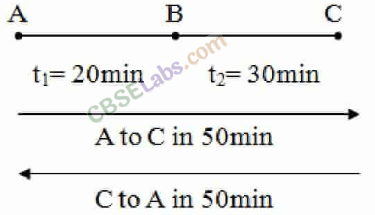Paheli is moving along a straight line with a fixed time period. So, her motion is rectilinear and periodic both.

Question 6.
Bholu and Golu are playing in a ground. They start running from the same point A in the ground and reach point B at the same time by following the paths marked 1 and 2 respectively as shown in figure. Which of the following is/are true for the given situation ?As compared to Golu, Bholu covers a
(a) longer distance but with a lower speed
(b) longer distance with a higher speed
(c) shorter distance with a lower speed
(d) shorter distance with a higher speed.
Solution:
(b) : Path 1 is longer than path 2 but Golu and Bholu both have reached point B at the same time, so Bholu’s speed is more than Golu’s speed.

Question 7.
Four pieces of wooden sticks A, B, C and D are placed along the length of 30 cm long scale as shown in figure. Which one of them is 3.4 cm in length?(a) A
(b) B
(c) C
(d) D
Solution:
From the given figure, we can observe that stick C lies between 10.4 cm and 7 cm. So, its length is (10.4 – 7) cm = 3.4 cm.

Question 8.
Which of the following figure shows the correct placement of a block along a scale for measuring its length?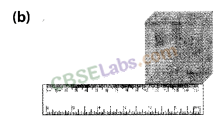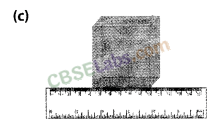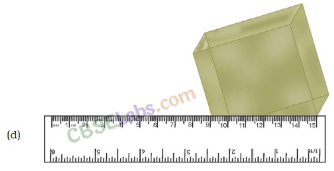Solution:
(c): Object should be placed on a scale such that its ends should come in between the readings marked on the scale.

Question 9.
You are provided three scales A, B and C as shown in figure to measure a length of 10 cm.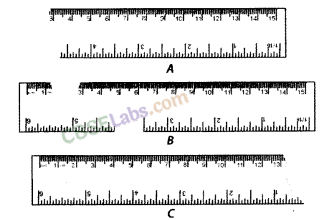For the correct measurement of the length you will use the scale
(a) A only
(b) B only
(c) C only
(d) Any of the three Scales.
Solution:
(d): Length of 10 cm is available in all the three scales A, B and C. So, any of the three scales’ can be used.
Correct the following.

Question 10.
(i) The motion of a swing is an example of rectilinear motion.
(ii) 1m = 1000cm
Solution:
(i) The motion of a swing is an example of periodic motion as it repeats its motion after a fixed interval of time.
(ii) 1 m = 100 cm. Each metre (m) is divided into 100 equal divisions, called centimetre (cm).

Question 11.
Fill in the blanks.
(i) Motion of an object or a part of it around a fixed point is known as_______ motion.
(ii) A body repeating its motion after certain interval of time is in_______ motion.
(iii) In rectilinear motion, object moves a______ line______.
(iv) SI unit of length is_______.
Solution:
(i) Circular
(ii) periodic
(iii) along; straight
(iv) metre

Question 12.
Write one example for each of the following type of motion.
(i) Rectilinear
(ii) Circular
(iii) Periodic
(iv) Circular and periodic
Solution:
(i) An apple falling vertically downwards.
(ii) The motion of a point marked on the hands of a clock.
(iii) Motion of a child on a swing.
(iv) Blades of a rotating fan.

Question 13.The photograph given in figure shows a section of a grille made up of straight and curved iron bars. How would you measure the length of the bars of this section, so that the payment could be made to the contractor?
Solution:
As the grille is made up of straight and curved iron bars, so the length of the bars can be measured with the help of thread which can be further measured using a scale.

Question 14.
Identify the different types of motion in the following word diagram given.

 Y O U N G C C N T E R L E V E L p I E E A R A L L O T o P P E A I N O T E P A D N E C K O W O N E w I Y Z s E I E V O R L O A D w P T R G N I C E D R I L A Z H T O N G U E N A T X C R D E P T H G R O E Y C I R C U L A R R T L C C O P P E R T

Solution:Question 15.
Four children measure the length of a table which was about 2 m. Each of them used different ways to measure it.
(i) Sam measured it with a half metre long thread.
(ii)with a 15 cm scale from her geometry box.
(iii) Reena measured it using her hand span.
(iv) Salim measured it using a 5 m long measuring tape.
Which one of them would get the most accurate length? Give reason for your answer.
Solution:
Salim would get the most accurate length because’ he is using a 5 m long measuring tape which is longer than the table. So, he can measure the length of the table in one go accurately. While in the other cases the chance of making an error is higher due to multiple measurements. In case of Sam, only that lengths can be measured which are exact multiples of half a metre.

Question 16.
Match the events related to motion in column I with the types of motions given in column II.

 Column 1 Column 11 (a) A moving wheel of a sewing machine (i) Circular motion (b) Movement of tip of the minute hand of a clock in one hour (ii) Rotational motion (0 A moving swing (iii) Periodic motion

(a) – (ii)
(b) – (i)
(c) – (iii)

Question 17.
While travelling in a train, it appears that the trees near the track are moving whereas co-passengers appear to be stationary. Explain the reason.
Solution:
While sitting in a moving train, when we look outside the window, the trees appear to be moving in opposite direction because their position is changing with respect to us. On the other hand the position of co-passengers is not changing with respect to us, hence they appear to be stationary.

Question 18.
How are the motions of a wheel of a moving bicycle and a mark on the blade of a moving electric fan different? Explain.
Solution:
The wheel of a moving bicycle depicts circular as well as rectilinear motion. Wheels of bicycle rotate on the ground which in turn moves the bicycle forward. A blade of a moving electric fan shows only circular motion as it is not moving from one place to another.

Question 19.
Three students measured the length of a corridor and reported their measurements. The values of their measurements were different.
What could be the reason for difference in their measurements? (Mention any three)
Solution:
Some of the reasons for difference in their measurement could be
(i) Different measuring devices were used.
(ii) The smallest length that could be _ measured by different devices may be different.
(iii) Measurement may not be along the shortest length in all three cases.
(iv) The end of the corridor may not be easily accessible.
(v) The measuring devices may be faulty (not standardised).

Question 20.
Boojho was riding in his bicycle along a straight road. He classified the motion of various parts of the bicycle as
(i) rectilinear motion,
(ii) circular motion and
(iii) both rectilinear as well as circular motion. Can you list one part of the bicycle for each type of motion? Support your answer with reason.
Solution:
(i) Rectilinear motion: Handle bar or seat of the bicycle has rectilinear motion only. It moves in a straight line as the wheels of bicycle move forward.
(ii) Circular motion: Pedal of the bicycle is having circular motion only as it rotates with its shaft but does not move from its place.
(iii) Rectilinear and circular motion: Wheel of the bicycle is having both circular and rectilinear motion. Wheels rotate on their ‘ shafts as well as move forward on the ground.

We hope the NCERT Exemplar Class 6 Science Chapter 10 Motion and Measurement of Distances will help you. If you have any query regarding NCERT Exemplar Class 6 Science Solutions Chapter 10 Motion and Measurement of Distances, drop a comment below and we will get back to you at the earliest.